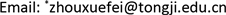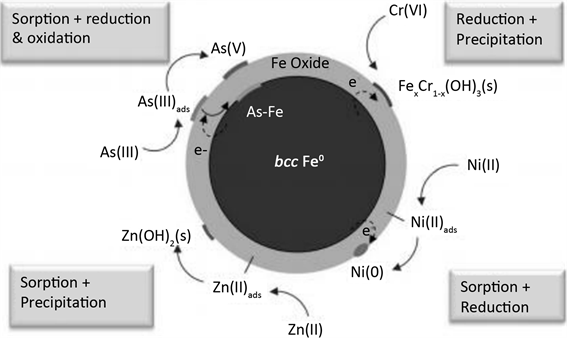﻿ 纳米零价铁对污染物去除作用的机理研究 Study on Mechanism of Nano-Zero-Valent Iron Removal of Pollutants

Vol. 09  No. 02 ( 2019 ), Article ID: 29872 , 5 pages
10.12677/AEP.2019.92032

Study on Mechanism of Nano-Zero-Valent Iron Removal of Pollutants

Huichao Guo, Jiabin Chen, Xuefei Zhou*

State Key Laboratory of Pollution Control and Resource Reuse, Tongji University, ShanghaiReceived: Apr. 2nd, 2019; accepted: Apr. 17th, 2019; published: Apr. 24th, 2019ABSTRACT

Nano-zero-valent iron has a lower standard electromotive force and nano-size, which greatly increases the active reaction sites and active adsorption sites on the surface, and the rate of reduction and degradation of pollutants is much higher than that of ordinary zero-valent iron materials. This paper reviews the removal mechanism of organic zero pollution and heavy metals by nano-zero-valent iron particles. The purpose is to provide reference and ideas for in-depth research in this field.

Keywords:Nano-Zero-Valent Iron, Pollutants, Mechanism1. 引言

2. 纳米零价铁与有机污染物

$\text{RCl}+\text{Fe}+{\text{H}}^{+}\to \text{RH}+{\text{Fe}}^{2+}+{\text{Cl}}^{-}$

$\text{Fe}+2{\text{H}}_{2}\text{O}\to {\text{Fe}}^{2+}+{\text{H}}_{2}+2{\text{OH}}^{-}$

$\text{RCl}+\text{Fe}+{\text{H}}^{+}\to \text{RH}+{\text{Fe}}^{\text{2}+}+{\text{Cl}}^{-}$

$\text{RCl}+{\text{2Fe}}^{\text{2}+}+{\text{H}}^{+}\to \text{RH}+{\text{2Fe}}^{\text{3}+}+{\text{Cl}}^{-}$

$\text{RCl}+{\text{H}}_{\text{2}}\to \text{RH}+{\text{H}}^{+}+{\text{Cl}}^{-}$Figure 1. Reaction mechanism of nano-zero-valent iron with chlorine-containing organic pollutants

3. 纳米零价铁与重金属元素Table 1. Comparison of nano-zero-valent iron removal of heavy metals 

1) 吸附–还原作用，适用于标准电极电势高于 $\text{Fe}\left(\text{Fe}\to {\text{Fe}}^{\text{2}+}+{\text{2e}}^{-},\text{\hspace{0.17em}}\text{E}0=-0.\text{41}\text{\hspace{0.17em}}\text{V}\right)$ 的重金属阳离子，即理论上可以被铁还原，如Cu2+、Ni2+、Pb2+等 。这些阳离子将首先被纳米零价铁颗粒吸附于其氧化壳上，再通过与零价铁核进行电子交换后被还原为零价重金属并固定于纳米零价铁颗粒上。Zhang  的研究表明，纳米零价铁表面有50%的Ni2+在3小时候逐步被还原为Ni。这类反应的方程式可以概括为：

$\text{FeOH}+{\text{M}}^{\text{2}+}\to {\text{FeO-M}}^{+}+{\text{H}}^{+}$

${\text{FeO-M}}^{+}+{\text{H}}_{\text{2}}\text{O}\to \text{FeO-M-OH}+{\text{H}}^{+}$

${\text{FeO-M}}^{+}+\text{Fe}+{\text{H}}^{+}\to \text{FeOH-M}+{\text{Fe}}^{\text{2}+}$

2) 吸附–共沉淀，适用于标准电极电势低于 $\text{Fe}\left(\text{Fe}\to {\text{Fe}}^{\text{2}+}+{\text{2e}}^{-},\text{\hspace{0.17em}}\text{E}0=-0.\text{41}\text{\hspace{0.17em}}\text{V}\right)$ 的重金属阳离子，即理论上不可以被铁还原，如Ba2+，Zn2+，Cd2+等。这些阳离子将首先被纳米零价铁颗粒吸附于其氧化壳上，由于纳米零价铁不能还原这些阳离子反应，因此纳米零价铁将与水应生成大量OH，改变纳米零价铁颗粒表面的酸碱性质，最后这些重金属离子和这些OH结合形成共沉淀并固定于纳米零价铁颗粒上，其反应方程式为：

$\text{Fe}+{\text{2H}}_{\text{2}}\text{O}\to {\text{Fe}}^{\text{2}+}+{\text{H}}_{\text{2}}+{\text{2OH}}^{-}$

$\text{FeOH}+{\text{M}}^{\text{2}+}\to {\text{FeO-M}}^{+}+{\text{H}}^{+}$

${\text{FeO-M}}^{+}+{\text{OH}}^{-}\to \text{FeO-M-OH}$

3) 还原–共沉淀。纳米零价铁能迅速将毒性以及迁移能力远远大于Cr(III)的Cr(V)还原到Cr(III)，并将Cr(III)固定于纳米零价铁微粒表面。陈芳艳  等对纳米零价铁去Cr的动力学进行了研究，发现纳米零价铁还原Cr(V)的速率比普通零价铁提高了6倍。因此纳米零价铁不但降低了Cr的毒性也实现了对Cr的固定。其反应方程式为：

$\text{3Fe}+{\text{Cr}}_{\text{2}}{\text{O}}_{7}^{2-}+{\text{14H}}^{+}\to {\text{3Fe}}^{\text{2}+}+{\text{2Cr}}^{\text{3}+}+{\text{7H}}_{\text{2}}\text{O}$

4) 吸附–氧化–还原。纳米零价铁不但可以将As(V)还原成As(0)，As(III)，将As(III)还原成As(0)，也可以将As(III)氧化成As(V)。Ramos  等分别分析纳米零价铁与As(V)和As(III)的反应产物，发现76%的As(III)被吸附在纳米零价铁表面，11%和13%的As(V)分别被还原为As(III)和As(0)。另一方面，不同形态的砷元素结合在纳米零价铁颗粒不同部位。Ramos  等也认为As(0)存在于靠近纳米零价铁内层，As(V)存在纳米零价铁最外层，而As(III)则存在两者之间。Figure 2. Reaction mechanism of nano-zero-valent iron and heavy metal pollutants

4. 结论与展望

Study on Mechanism of Nano-Zero-Valent Iron Removal of Pollutants[J]. 环境保护前沿, 2019, 09(02): 220-224. https://doi.org/10.12677/AEP.2019.92032

1. 1. 李长嘉, 潘成忠, 雷宏军, 田培. 1992-2008年我国工业废水排放变化效应[J]. 环境科学研究, 2013, 26(5): 569-575.

2. 2. 樊绑棠. 环境化学[M]. 杭州: 浙江大学出版社, 1991.

3. 3. 李钰婷, 张亚雷, 代朝猛, 张伟贤. 纳米零价铁颗粒去除水中重金属的研究进展[J]. 环境化学, 2012, 31(9): 1349-1354.

4. 4. 陈冠兰, 陈银广. 低成本生物材料吸附六价铬研究进展[J]. 水处理技术, 2008, 34(12): 7-10.

5. 5. 闫研, 李建平, 赵志围. 超富集植物对重金属耐受和富集机制的研究进展[J]. 广西植物, 2008, 28(4): 505-510.

6. 6. Zhang, W.X. (2003) Nanoscale Iron Particles for Environmental Remediation: On Overview. Journal of Nanoparticle Research, 5, 323-332. https://doi.org/10.1023/A:1025520116015

7. 7. 徐新华, 卫建军, 汪大翚. Pd/Fe及纳米Pd/Fe对氯酚的脱氯研究[J]. 中国环境科学, 2004, 24(1): 76-80.

8. 8. 何小娟, 刘菲, 黄园英. 利用零价铁去除挥发性氯代脂肪烃的试验[J]. 环境科学, 2003, 24(1): 139-142.

9. 9. Li, X.Q. and Zhang, W.X. (2007) Sequestration of Metal Cations with Zerovalent Iron Nano-particles: A Study with High Resolution X-Ray Photoelectron Spectroscopy (HR-XPS). Journal of Physical Chemistry C, 111, 6939-6946. https://doi.org/10.1021/jp0702189

10. 10. Li, X.Q. and Zhang, W.X. (2006) Iron Nanoparticles: The Core-Shell Structure and Unique Properties for Ni(II) Sequestration. Langmuir, 22, 4638-4642. https://doi.org/10.1021/la060057k

11. 11. 陈芳艳, 唐玉斌, 吕锡武. 纳米零价铁对水中Cr(VI)的还原动力学研究[J]. 化学世界, 2007, 48(3): 144-147.

12. 12. Ramos Mauricio, A.V., Yan, W.L. and Li, X.Q. (2009) Simultaneous Oxidation and Reduction of Arsenic by Zero-Valent Iron Nanoparticles: Under-standing the Significance of the Core-Shell Structure. Journal of Physical Chemistry C, 113, 14-1-14594. https://doi.org/10.1021/jp9051837